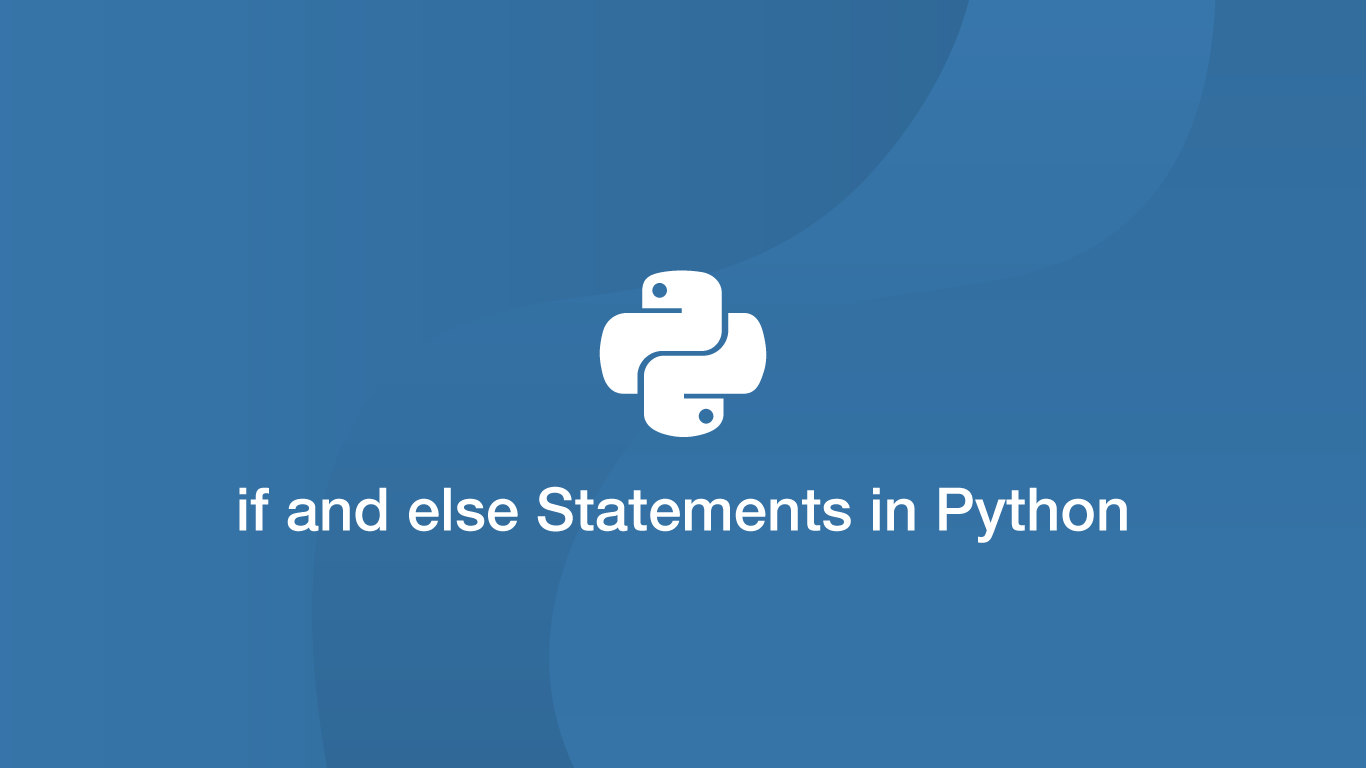# How to Use if and else Statements in Python`if` and `else` statements are a fundamental part of making programs in Python and any other procedural programming language. They allow us to determine the result of inputs and whether an output is displayed.

In this tutorial, we will go through how to use if, else and else if statements in Python.

## if Statement

Let's say we wanted to compare two values and `print` something if the comparison is `true`. We could achieve such a task using an `if` statement. In the example below, we are checking if `2` is greater than `1` and printing `true` if it is the case.

``````if 2 > 1:
print('true')
``````
``````true
``````

## Python Syntax

At this point, it is worth going over the syntax of python. If you are familiar with other procedural programming languages such as PHP you will have probably noticed that Python does not make use of `{}` (opening and closing curly brackets) - instead, it just uses a `:` (colon) to open statements. The reason for this is Python depends on indentation to determine what should be within a statement.

``````if 1 > 2:
print('true')
``````

The above statement will print nothing as `1` is not greater than two and `print('true'`) is within the `if` statement as it is indented with a `    ` (tab, or four space characters.)

``````if 1 > 2:
print('true')
``````
``````  File "<ipython-input-11-8fa672332a35>", line 2
print('true')
^
IndentationError: expected an indented block
``````

The above statement returns an error because no indentation was used.

``````if 1 > 2:
print('true')
print('outside of if statement')
``````
``````outside of if statement
``````

`outside of if statement` is printed because it is not indented and therefore outside of the `if` statement.

## else Statement

We can combine `else` with an `if` statement to do something if the `if` comparison is not true. `else` should be at the same indentation level as its `if` counterpart and must include an opening `:` (colon).

``````if 1 > 2:
print('true')
else:
print('not true')
``````
``````not true
``````

## elif Statement

If we need to perform a further comparison if an `if` statement is false we can use `elif`, which is shorthand for `elseif`. The syntax is exactly the same as an `if` statement.

``````if 1 > 2:
print('true')
elif 2 > 1:
print('true, two is greater than one')
``````
``````two is greater than one
``````

## Nesting if Statements

When nesting `if`, `else` and `elif` statements it is important to observe Pythons indentation of rules. Each time a statement is nested in must be tabbed in one more time than its parent.

``````if 2 > 1:
print('2 is greater than 1')
if 2 == 3:
print('2 equals 3')
elif 3 > 2:
print('3 is greater than 2')
if 1 == 1:
print('1 equals 1')
``````
``````2 is greater than 1
3 is greater than 2
1 equals 1
``````

## Conclusion

You now know how to use `if` statements in Python and how to combine them with `else` and `elif` statements. The indentation method of containing statements is cleaner than opening and closing brackets as seen in other languages so getting used to the Python syntax should be pretty straightforward.

#### Related Tutorials### How to Use Python List Comprehension

October 04, 2020### Python Assert Statement

September 30, 2020### How to use Python Try Except Block

June 14, 2021### How to Use For Loops in Python

September 02, 2020### How to Check if a String Contains a Substring in Python

September 21, 2020June 23, 2021